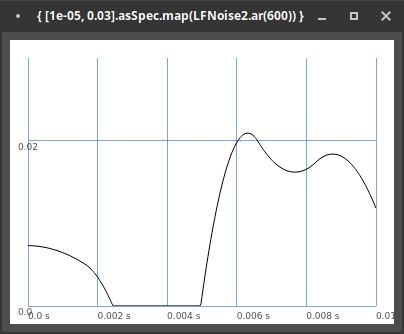hey, i thought it would be possible to rewrite this:

`coef = Array.fill(5, { [1e-05, 0.03].asSpec.map(LFNoise2.kr(3)) } );`

to that:

``````coef = Array.fill(5, { LFNoise2.kr(3).range(1e-05, 0.03) } );
``````

but its giving me different results. any ideas how to convert this using `.linlin` or `.range` instead of `.addSpec.map` thanksI think “how to convert” isn’t the first question.

Programming comes down to:

1. What are the inputs?
2. What is the output?
3. What’s happening to translate input into output? (For instance, in linlin and linexp, the inputs and output could be described in the same terms, but the math is different.)
• (3a. Are there any side effects? … but this question is about pure functions, so we don’t have to consider that right now.)

So, `map`:

• Inputs: A ControlSpec (receiver), and a number or signal – but, more specifically, check the help: “Maps and constrains a value between 0 and 1…” So the input is expected to be 0.0 – 1.0.
• Output: A number / signal mapped “to the range between minval and maxval.”
• Operations: Depend on the curve parameter.

0.0 – 1.0 → minval – maxval.

The signal input is itself the result of a method, `kr` – input: An LFO rate. Output: Here, the help only hints at the range: “quadratic interpolation means that the noise values can occasionally extend beyond the normal range of ±1.” Also `LFNoise2.kr.signalRange``\bipolar`.

So `map` expects 0.0 to 1.0, but receives -1.0 to 1.0 – well, that’s not going to work. To make it work, you need to convert the bipolar to a unipolar normal range.

``````coef = Array.fill(5, { [1e-05, 0.03].asSpec.map(LFNoise2.kr(3) * 0.5 + 0.5) } );
``````
• LFNoise2 = -1 to 1
• `* 0.5` = -0.5 to 0.5
• `+ 0.5` = 0 to 1

Meanwhile `ugen.range(lo, hi)` checks the UGen’s `signalRange`. For a bipolar UGen, -1.0 in → lo out, 1.0 in → hi out.

The takeaway is that in signal processing, signal ranges are extremely important. For every unit and every math operation, you should have an idea of the low and high bounds.

hjh

thank you very much for this detailed explanation.

during my ongoing research on stick-slip synthesis ive looked around for examples using the `Friction` uGen.
The only example i could find has been this one:

``````(
{
var sig, coef;

coef = Array.fill(5, { [1e-05, 0.03].asSpec.map(LFNoise2.kr(3)) } ).poll;
//coef = Array.fill(5, { [1e-05, 0.03].asSpec.map(LFNoise2.kr(3) * 0.5 + 0.5) } ).poll;
//coef = Array.fill(5, { LFNoise2.kr(3).linlin(-1, 1, 1e-05, 0.03) } ).poll;

sig = LFTri.ar(50);
sig = Friction.ar(sig, friction: coef, mass: coef * 3e4);

Splay.ar(sig);
}.play
)
``````

The result hasnt been too impressive to me but i liked the glitchy quality of it and in addition a method was used `.asSpec.map` which i havent been familiar with before. So i thought of converting it to something i can already deal with (and made some mistakes on the way not taking into account the output ranges of the `LFNoise2`) and was wondering why its not giving me the same results. In general with all these “converting” questions im trying to transfer something new to something i already know.
But is there possibly a mistake in the example which i found using `coef = Array.fill(5, { [1e-05, 0.03].asSpec.map(LFNoise2.kr(3)) } ).poll;` or is this more on purpose? the result is pretty different when taking into account the output ranges of `LFNoise2`. This was leading to more confusion then it was helping me out to generalize things in relation to Stick-Slip Sythesis.

I think it’s a mistake.

The ControlSpec will clip negative values from the LFNoise:

``````{ [1e-05, 0.03].asSpec.map(LFNoise2.ar(600)) }.plot(minval: 1e-05, maxval: 0.03)
``````I can’t imagine that’s deliberate. If you’re using a cubic-interpolation noise generator, presumably you want smooth curves between control points. Clipping completely destroys the shape.

It’s more important to understand what you want and write code for that. “I found this example online” may be a good learning experience, or it could be a bad one, and you might not know which is which until you work through what the code means and determine whether it lines up with what you want.

hjh

thank you very much